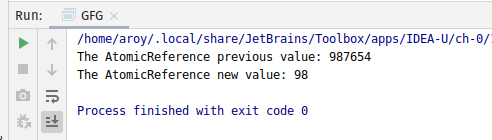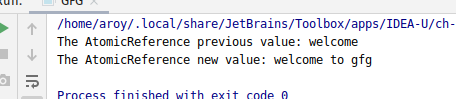Related Articles
AtomicReference getAndUpdate() method in Java with Examples
• Last Updated : 03 Jan, 2020

The getAndUpdate() method of a AtomicReference class is used to atomically updates which updates the current value of the AtomicReference by applying the specified updateFunction operation on the current value. It takes an object of updateFunction interface as its parameter and applies the operation specified in the object to the current value. It returns the previous value.

Syntax:

```public final V getAndUpdate(UnaryOperator<V> updateFunction)
```

Parameters: This method accepts updateFunction which is a side-effect-free function.

Return value: This method returns the prevoius value.

Below programs illustrate the getAndUpdate() method:
Program 1:

 `// Java program to demonstrate``// AtomicReference.getAndUpdate() method`` ` `import` `java.util.concurrent.atomic.AtomicReference;``import` `java.util.function.UnaryOperator;`` ` `public` `class` `GFG {``    ``public` `static` `void` `main(String args[])``    ``{`` ` `        ``// AtomicReference with value``        ``AtomicReference ref``            ``= ``new` `AtomicReference<>(``987654``);`` ` `        ``// Declaring the updateFunction``        ``// applying function``        ``UnaryOperator twoDigits``            ``= (v)``            ``-> v.toString()``                   ``.substring(``0``, ``2``);`` ` `        ``// apply getAndUpdate()``        ``int` `value = ref.getAndUpdate(twoDigits);`` ` `        ``// print AtomicReference``        ``System.out.println(``            ``"The AtomicReference previous value: "``            ``+ value);``        ``System.out.println(``            ``"The AtomicReference new value: "``            ``+ ref.get());``    ``}``}`
Output:Program 2:

 `// Java program to demonstrate``// AtomicReference.getAndUpdate() method`` ` `import` `java.util.concurrent.atomic.*;``import` `java.util.function.UnaryOperator;`` ` `public` `class` `GFG {``    ``public` `static` `void` `main(String args[])``    ``{`` ` `        ``// AtomicReference with value``        ``AtomicReference ref``            ``= ``new` `AtomicReference<>(``"welcome"``);`` ` `        ``// Declaring the updateFunction``        ``// applying function``        ``UnaryOperator twoDigits``            ``= (v) -> v + ``" to gfg"``;`` ` `        ``// apply getAndUpdate()``        ``String value``            ``= ref.getAndUpdate(twoDigits);`` ` `        ``// print AtomicReference``        ``System.out.println(``            ``"The AtomicReference previous value: "``            ``+ value);``        ``System.out.println(``            ``"The AtomicReference new value: "``            ``+ ref.get());``    ``}``}`
Output:Attention reader! Don’t stop learning now. Get hold of all the important Java Foundation and Collections concepts with the Fundamentals of Java and Java Collections Course at a student-friendly price and become industry ready. To complete your preparation from learning a language to DS Algo and many more,  please refer Complete Interview Preparation Course.

My Personal Notes arrow_drop_up